# Data Representation

Feb. 16, 2015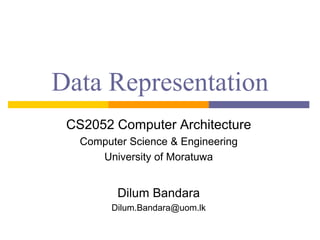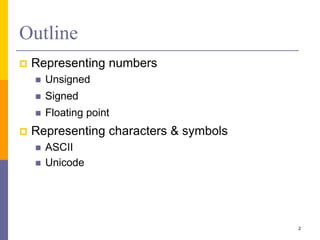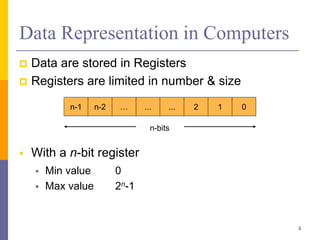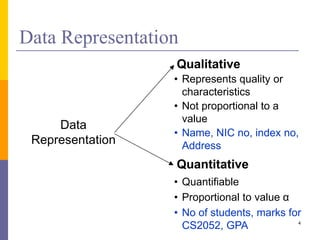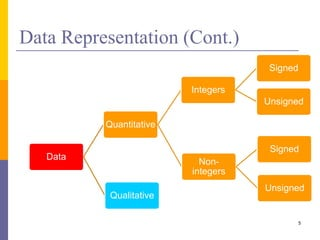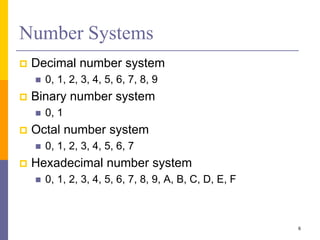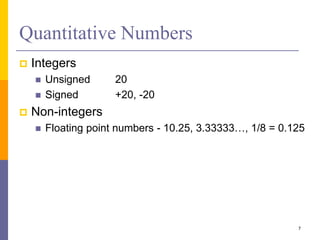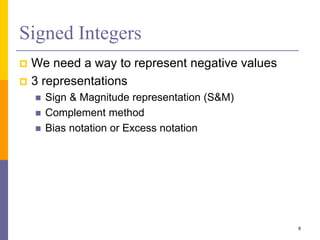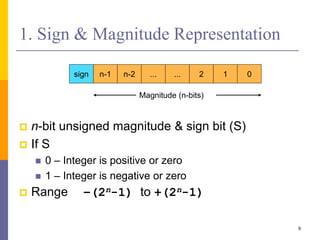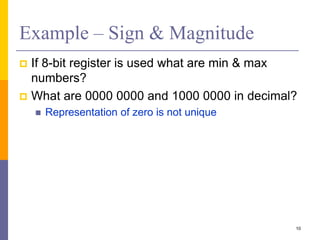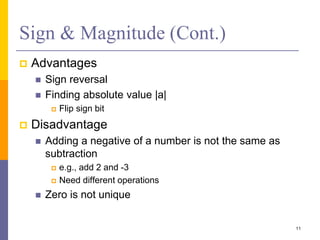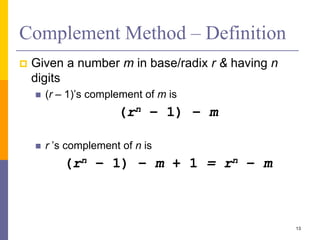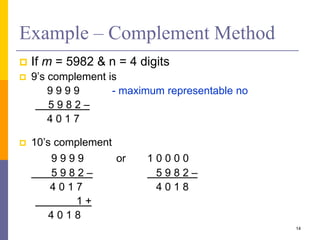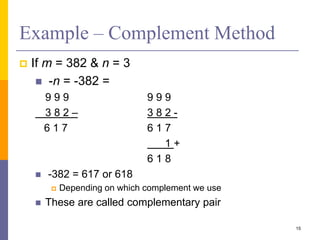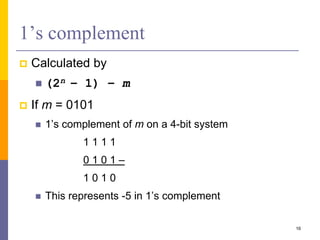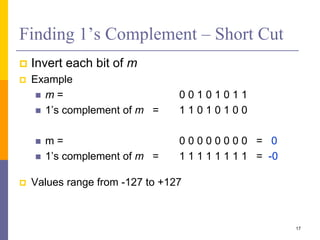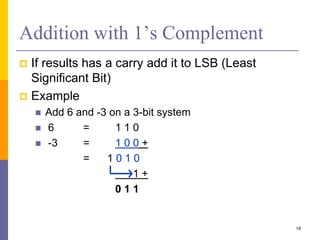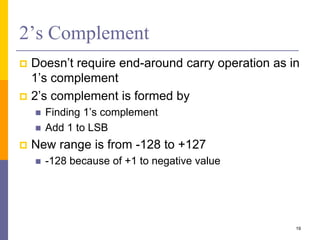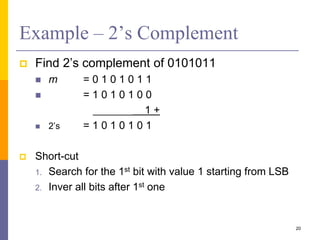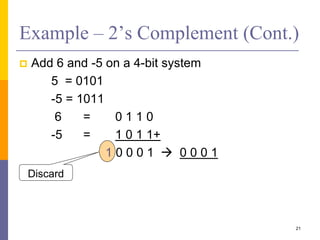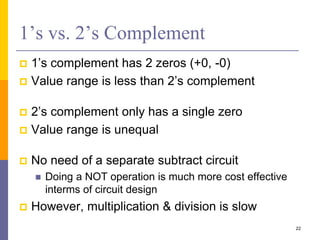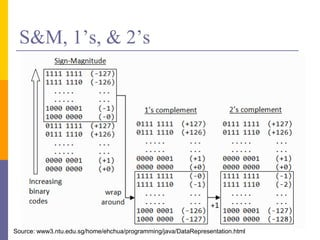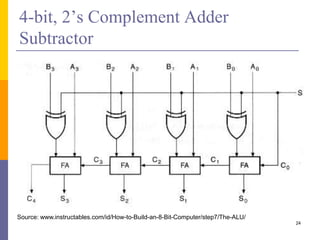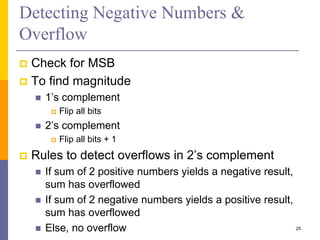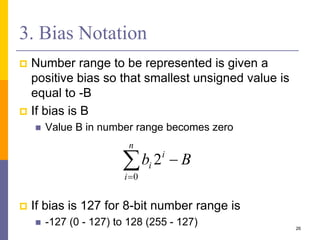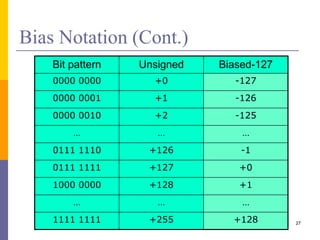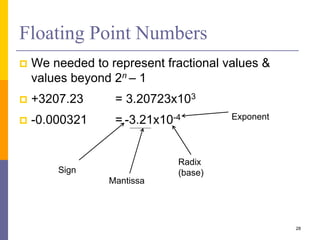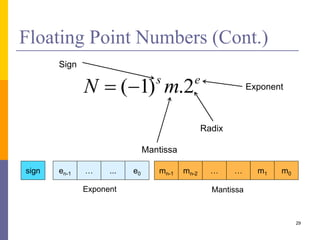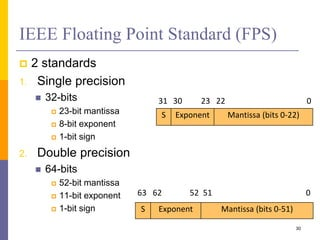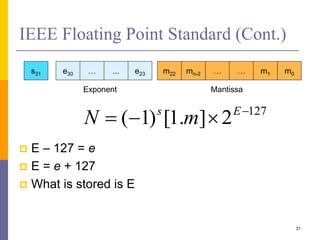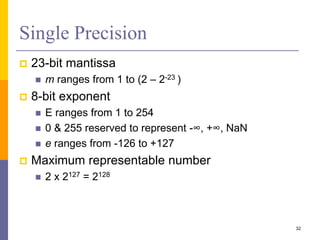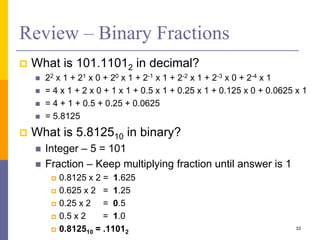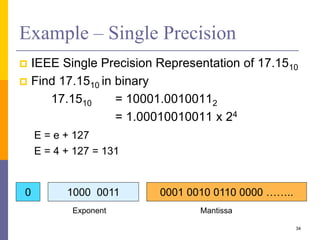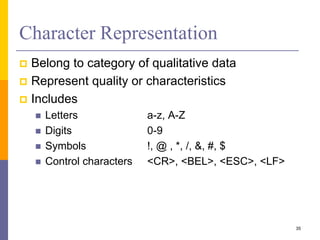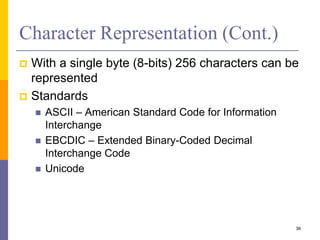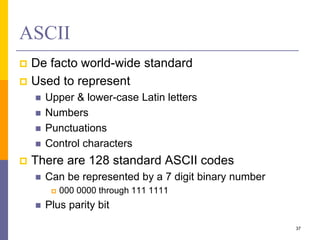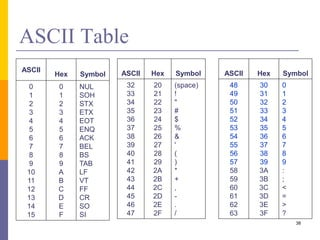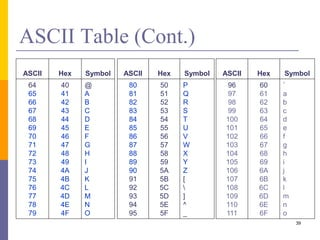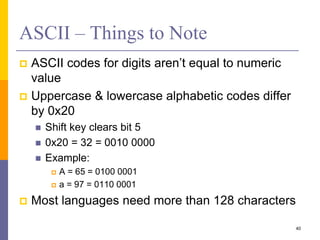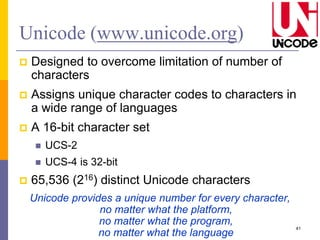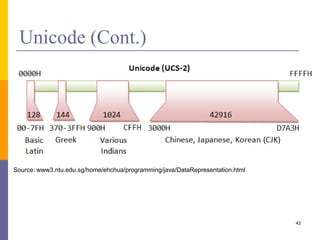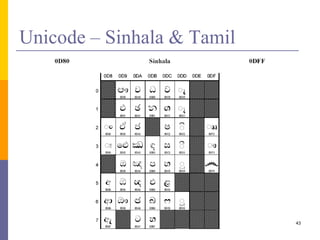1 of 43

### Data Representation

1. Data Representation CS2052 Computer Architecture Computer Science & Engineering University of Moratuwa Dilum Bandara Dilum.Bandara@uom.lk
2. Outline  Representing numbers  Unsigned  Signed  Floating point  Representing characters & symbols  ASCII  Unicode 2
3. Data Representation in Computers  Data are stored in Registers  Registers are limited in number & size  With a n-bit register  Min value 0  Max value 2n-1 3 n-1 n-2 ...… ... 2 01 n-bits
4. 4 Data Representation Data Representation • Represents quality or characteristics • Not proportional to a value • Name, NIC no, index no, Address Qualitative Quantitative • Quantifiable • Proportional to value α • No of students, marks for CS2052, GPA
5. Data Representation (Cont.) 5 Data Quantitative Integers Signed Unsigned Non- integers Signed Unsigned Qualitative
6. Number Systems  Decimal number system  0, 1, 2, 3, 4, 5, 6, 7, 8, 9  Binary number system  0, 1  Octal number system  0, 1, 2, 3, 4, 5, 6, 7  Hexadecimal number system  0, 1, 2, 3, 4, 5, 6, 7, 8, 9, A, B, C, D, E, F 6
7. Quantitative Numbers  Integers  Unsigned 20  Signed +20, -20  Non-integers  Floating point numbers - 10.25, 3.33333…, 1/8 = 0.125 7
8. Signed Integers  We need a way to represent negative values  3 representations  Sign & Magnitude representation (S&M)  Complement method  Bias notation or Excess notation 8
9. 1. Sign & Magnitude Representation  n-bit unsigned magnitude & sign bit (S)  If S  0 – Integer is positive or zero  1 – Integer is negative or zero  Range –(2n-1) to +(2n-1) 9 sign n-1 ...n-2 ... 2 01 Magnitude (n-bits)
10. Example – Sign & Magnitude  If 8-bit register is used what are min & max numbers?  What are 0000 0000 and 1000 0000 in decimal?  Representation of zero is not unique 10
11. Sign & Magnitude (Cont.)  Advantages  Sign reversal  Finding absolute value |a|  Flip sign bit  Disadvantage  Adding a negative of a number is not the same as subtraction  e.g., add 2 and -3  Need different operations  Zero is not unique 11
12. 2. Complement Method  Base = Radix  Radix r system means r number of symbols  e.g., binary numbers have symbols 0, 1  2 types  r’s complement  (r – 1)’s complement  Where r is radix (base) of number system  Examples  Decimal 9’s & 10’s complement  Binary 1’s & 2’s complement 12
13. Complement Method – Definition  Given a number m in base/radix r & having n digits  (r – 1)’s complement of m is (rn – 1) – m  r ’s complement of n is (rn – 1) – m + 1 = rn – m 13
14. Example – Complement Method  If m = 5982 & n = 4 digits  9’s complement is 9 9 9 9 - maximum representable no 5 9 8 2 – 4 0 1 7  10’s complement 9 9 9 9 or 1 0 0 0 0 5 9 8 2 – 5 9 8 2 – 4 0 1 7 4 0 1 8 1 + 4 0 1 8 14
15. Example – Complement Method  If m = 382 & n = 3  -n = -382 = 9 9 9 9 9 9 3 8 2 – 3 8 2 - 6 1 7 6 1 7 1 + 6 1 8  -382 = 617 or 618  Depending on which complement we use  These are called complementary pair 15
16. 1’s complement  Calculated by  (2n – 1) – m  If m = 0101  1’s complement of m on a 4-bit system 1 1 1 1 0 1 0 1 – 1 0 1 0  This represents -5 in 1’s complement 16
17. Finding 1’s Complement – Short Cut  Invert each bit of m  Example  m = 0 0 1 0 1 0 1 1  1’s complement of m = 1 1 0 1 0 1 0 0  m = 0 0 0 0 0 0 0 0 = 0  1’s complement of m = 1 1 1 1 1 1 1 1 = -0  Values range from -127 to +127 17
18. Addition with 1’s Complement  If results has a carry add it to LSB (Least Significant Bit)  Example  Add 6 and -3 on a 3-bit system  6 = 1 1 0  -3 = 1 0 0 + = 1 0 1 0 1 + 0 1 1 18
19. 2’s Complement  Doesn’t require end-around carry operation as in 1’s complement  2’s complement is formed by  Finding 1’s complement  Add 1 to LSB  New range is from -128 to +127  -128 because of +1 to negative value 19
20. Example – 2’s Complement  Find 2’s complement of 0101011  m = 0 1 0 1 0 1 1  = 1 0 1 0 1 0 0 ________ 1 +  2’s = 1 0 1 0 1 0 1  Short-cut 1. Search for the 1st bit with value 1 starting from LSB 2. Inver all bits after 1st one 20
21. Example – 2’s Complement (Cont.)  Add 6 and -5 on a 4-bit system 5 = 0101 -5 = 1011 6 = 0 1 1 0 -5 = 1 0 1 1+ 1 0 0 0 1  0 0 0 1 21 Discard
22. 1’s vs. 2’s Complement  1’s complement has 2 zeros (+0, -0)  Value range is less than 2’s complement  2’s complement only has a single zero  Value range is unequal  No need of a separate subtract circuit  Doing a NOT operation is much more cost effective interms of circuit design  However, multiplication & division is slow 22
23. S&M, 1’s, & 2’s 23 Source: www3.ntu.edu.sg/home/ehchua/programming/java/DataRepresentation.html
24. 4-bit, 2’s Complement Adder Subtractor 24 Source: www.instructables.com/id/How-to-Build-an-8-Bit-Computer/step7/The-ALU/
25. Detecting Negative Numbers & Overflow  Check for MSB  To find magnitude  1’s complement  Flip all bits  2’s complement  Flip all bits + 1  Rules to detect overflows in 2’s complement  If sum of 2 positive numbers yields a negative result, sum has overflowed  If sum of 2 negative numbers yields a positive result, sum has overflowed  Else, no overflow 25
26. 3. Bias Notation  Number range to be represented is given a positive bias so that smallest unsigned value is equal to -B  If bias is B  Value B in number range becomes zero  If bias is 127 for 8-bit number range is  -127 (0 - 127) to 128 (255 - 127) 26   n i i i Bb 0 2
27. Bias Notation (Cont.) 27 Bit pattern Unsigned Biased-127 0000 0000 +0 -127 0000 0001 +1 -126 0000 0010 +2 -125 … … … 0111 1110 +126 -1 0111 1111 +127 +0 1000 0000 +128 +1 … … … 1111 1111 +255 +128
28. Floating Point Numbers  We needed to represent fractional values & values beyond 2n – 1  +3207.23 = 3.20723x103  -0.000321 = -3.21x10-4 28 Sign Mantissa Radix (base) Exponent
29. Floating Point Numbers (Cont.) 29 es mN 2.)1( Sign Mantissa Radix Exponent sign en-1 ...… e0 … m0m1mn-1 …mn-2 MantissaExponent
30. IEEE Floating Point Standard (FPS)  2 standards 1. Single precision  32-bits  23-bit mantissa  8-bit exponent  1-bit sign 2. Double precision  64-bits  52-bit mantissa  11-bit exponent  1-bit sign 30 31 30 23 22 0 S Exponent Mantissa (bits 0-22) 63 62 52 51 0 S Exponent Mantissa (bits 0-51)
31. IEEE Floating Point Standard (Cont.)  E – 127 = e  E = e + 127  What is stored is E 31 s31 e30 ...… e23 … m0m1m22 …mn-2 MantissaExponent 127 2].1[)1(   Es mN
32. Single Precision  23-bit mantissa  m ranges from 1 to (2 – 2-23 )  8-bit exponent  E ranges from 1 to 254  0 & 255 reserved to represent -∞, +∞, NaN  e ranges from -126 to +127  Maximum representable number  2 x 2127 = 2128 32
33. Review – Binary Fractions  What is 101.11012 in decimal?  22 x 1 + 21 x 0 + 20 x 1 + 2-1 x 1 + 2-2 x 1 + 2-3 x 0 + 2-4 x 1  = 4 x 1 + 2 x 0 + 1 x 1 + 0.5 x 1 + 0.25 x 1 + 0.125 x 0 + 0.0625 x 1  = 4 + 1 + 0.5 + 0.25 + 0.0625  = 5.8125  What is 5.812510 in binary?  Integer – 5 = 101  Fraction – Keep multiplying fraction until answer is 1  0.8125 x 2 = 1.625  0.625 x 2 = 1.25  0.25 x 2 = 0.5  0.5 x 2 = 1.0  0.812510 = .11012 33
34. Example – Single Precision  IEEE Single Precision Representation of 17.1510  Find 17.1510 in binary 17.1510 = 10001.00100112 = 1.00010010011 x 24 E = e + 127 E = 4 + 127 = 131 34 0 1000 0011 0001 0010 0110 0000 …….. MantissaExponent
35. Character Representation  Belong to category of qualitative data  Represent quality or characteristics  Includes  Letters a-z, A-Z  Digits 0-9  Symbols !, @ , *, /, &, #, \$  Control characters <CR>, <BEL>, <ESC>, <LF> 35
36. Character Representation (Cont.)  With a single byte (8-bits) 256 characters can be represented  Standards  ASCII – American Standard Code for Information Interchange  EBCDIC – Extended Binary-Coded Decimal Interchange Code  Unicode 36
37. ASCII  De facto world-wide standard  Used to represent  Upper & lower-case Latin letters  Numbers  Punctuations  Control characters  There are 128 standard ASCII codes  Can be represented by a 7 digit binary number  000 0000 through 111 1111  Plus parity bit 37
38. ASCII Hex Symbol 32 33 34 35 36 37 38 39 40 41 42 43 44 45 46 47 20 21 22 23 24 25 26 27 28 29 2A 2B 2C 2D 2E 2F (space) ! " # \$ % & ' ( ) * + , - . / 38 ASCII Table ASCII Hex Symbol 0 1 2 3 4 5 6 7 8 9 10 11 12 13 14 15 0 1 2 3 4 5 6 7 8 9 A B C D E F NUL SOH STX ETX EOT ENQ ACK BEL BS TAB LF VT FF CR SO SI ASCII Hex Symbol 48 49 50 51 52 53 54 55 56 57 58 59 60 61 62 63 30 31 32 33 34 35 36 37 38 39 3A 3B 3C 3D 3E 3F 0 1 2 3 4 5 6 7 8 9 : ; < = > ?
39. ASCII Hex Symbol 80 81 82 83 84 85 86 87 88 89 90 91 92 93 94 95 50 51 52 53 54 55 56 57 58 59 5A 5B 5C 5D 5E 5F P Q R S T U V W X Y Z [ ] ^ _ 39 ASCII Table (Cont.) ASCII Hex Symbol 64 65 66 67 68 69 70 71 72 73 74 75 76 77 78 79 40 41 42 43 44 45 46 47 48 49 4A 4B 4C 4D 4E 4F @ A B C D E F G H I J K L M N O ASCII Hex Symbol 96 97 98 99 100 101 102 103 104 105 106 107 108 109 110 111 60 61 62 63 64 65 66 67 68 69 6A 6B 6C 6D 6E 6F ` a b c d e f g h i j k l m n o
40. ASCII – Things to Note  ASCII codes for digits aren’t equal to numeric value  Uppercase & lowercase alphabetic codes differ by 0x20  Shift key clears bit 5  0x20 = 32 = 0010 0000  Example:  A = 65 = 0100 0001  a = 97 = 0110 0001  Most languages need more than 128 characters 40
41. Unicode (www.unicode.org)  Designed to overcome limitation of number of characters  Assigns unique character codes to characters in a wide range of languages  A 16-bit character set  UCS-2  UCS-4 is 32-bit  65,536 (216) distinct Unicode characters Unicode provides a unique number for every character, no matter what the platform, no matter what the program, no matter what the language 41
42. Unicode (Cont.) 42 Source: www3.ntu.edu.sg/home/ehchua/programming/java/DataRepresentation.html
43. Unicode – Sinhala & Tamil 43